# Please answer the following questions using exponential and logarithmic models. 4) A wooden artif...

4) A wooden artifact from an archaeological dig contains 70 percent of the Carbon-14 that is present in living trees. To the nearest year, about how many years old is the artifact? (The half-life of Carbon-14 is 5730 years.) In years

5) A tumor is injected with 0.5 grams of Iodine-125, which has a decay rate of 1.15% per day. Write an exponential model representing the amount of Iodine-125 remaining in the tumor after t days. Then use the formula to find the amount of Iodine-125 that would remain in the tumor after 60 day

6) A doctor prescribes 100 milligrams of a therapeutic drug that decays by about 20% each hour. To the nearest hour, what is the half-life of the drug?

7) A tumor is injected with 0.4 grams of Iodine-125, which has a decay rate of 1.15% per day. To the nearest day, how long will it take for half of the Iodine-125 to decay? How many Days?

8) A tumor is injected with 0.4 grams of Iodine-125, which has a decay rate of 1.15% per day. Write an exponential model representing the number of grams f of Iodine-125 remaining in the tumor after t days.

f(t)=?

Then use the formula to find the amount of Iodine-125 that would remain in the tumor after 30 days. (Round your answer to four decimal places.) In grams

9) The half-life of Erbium-171 is 7.5 hours. What is the hourly decay rate? Express the result to four decimal places.

Express the hourly decay rate as a percentage to two decimal places. In percent %

4. Let the initial amount of Carbon be N0

Amount of carbon after t years = N0 e-kt

Given, half life = 5730 years

So, N0/2 = N0 e-k(5730)

=> 1/2 = e-5730k

=> ln (e-5730k) = ln (1/2)

=> -5730k = ln(1/2)

=> k = 0.000121

Let the artifact be t years old

0.7N0 = N0 e-0.000121t

=> 0.7 = e-0.000121t

=> ln (e-0.000121t) = ln 0.7

=> -0.000121t = ln (0.7)

=> t = 2947.73 years

5. 1-day decay percent = 1.15% = 0.0115

For x percent decrease , decay factor g is given by

g = 1 - 0.0115 = 0.9885

Initial amount = 0.5 grams

Amount of Iodine after t days = Initial Amount * (1-day decay factor)t

=> A(t) = 0.5(0.9885)t

Amount of Iodine after 60 days = 0.5(0.9885)60 = 0.2498 gm

6. 1-hour decay percent = 20% = 0.2

For x percent decrease , decay factor g is given by

g = 1 - 0.2 = 0.8

Let the initial amount be No

Amount of drug after t hours = No (0.8)t

Let the half life be n hours

So, No/2 = No (0.8)n

=> (0.8)n = 0.5

=> ln (0.8)n = ln 0.5

=> n ln 0.8 = ln 0.5

=> n = 3 hours

So, the half life of the drug is 3 hours

#### Earn Coin

Coins can be redeemed for fabulous gifts.

Similar Homework Help Questions
• ### Modeling Exponential Growth and Decay Madeleine Younes A wooden artifact from an archaeological dig contains 76...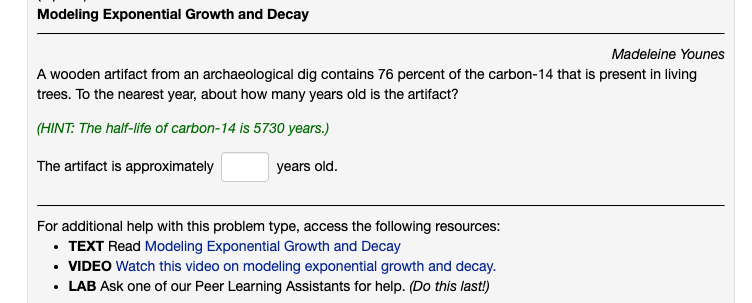Modeling Exponential Growth and Decay Madeleine Younes A wooden artifact from an archaeological dig contains 76 percent of the carbon-14 that is present in living trees. To the nearest year, about how many years old is the artifact? (HINT: The half-life of carbon-14 is 5730 years.) The artifact is approximately years old. For additional help with this problem type, access the following resources: • TEXT Read Modeling Exponential Growth and Decay • VIDEO Watch this video on modeling exponential growth...

• ### 54. Using the scenario below, model the situation using an exponential function and a base of...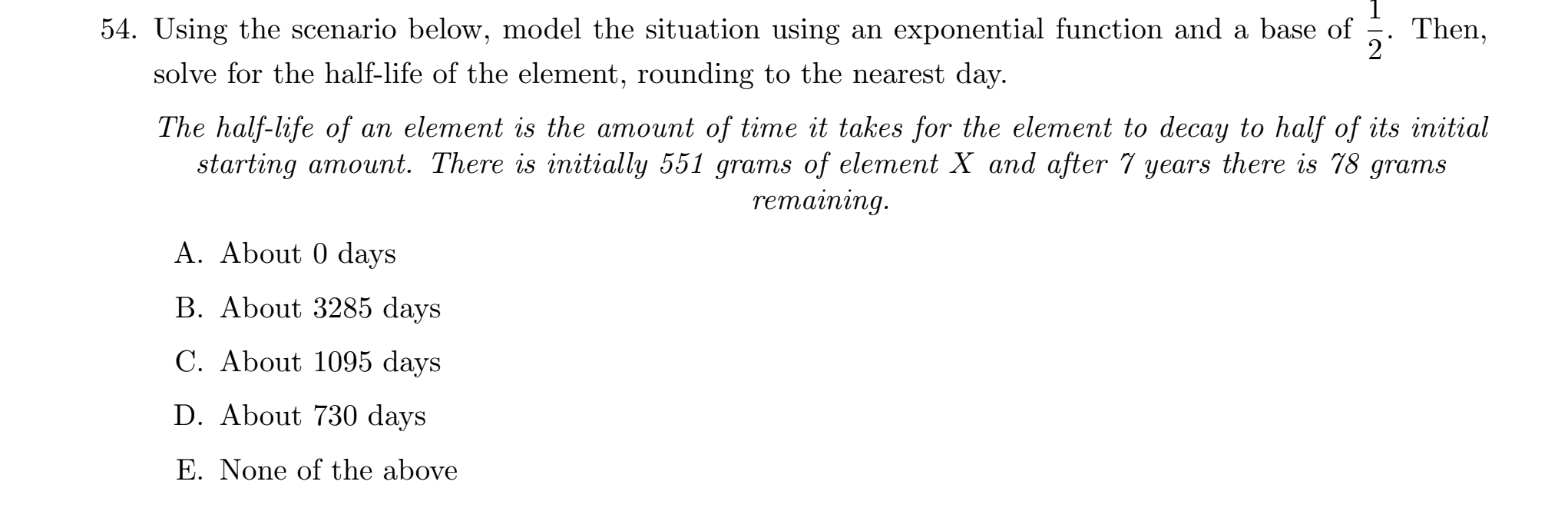54. Using the scenario below, model the situation using an exponential function and a base of Then, 2 solve for the half-life of the element, rounding to the nearest day. The half-life of an element is the amount of time it takes for the element to decay to half of its initial starting amount. There is initially 551 grams of element X and after 5 years there is 78 grams remaining. A. About 0 days B. About 3285 days C....

• ### 1 55. Using the scenario below, model the situation using an exponential function and a base...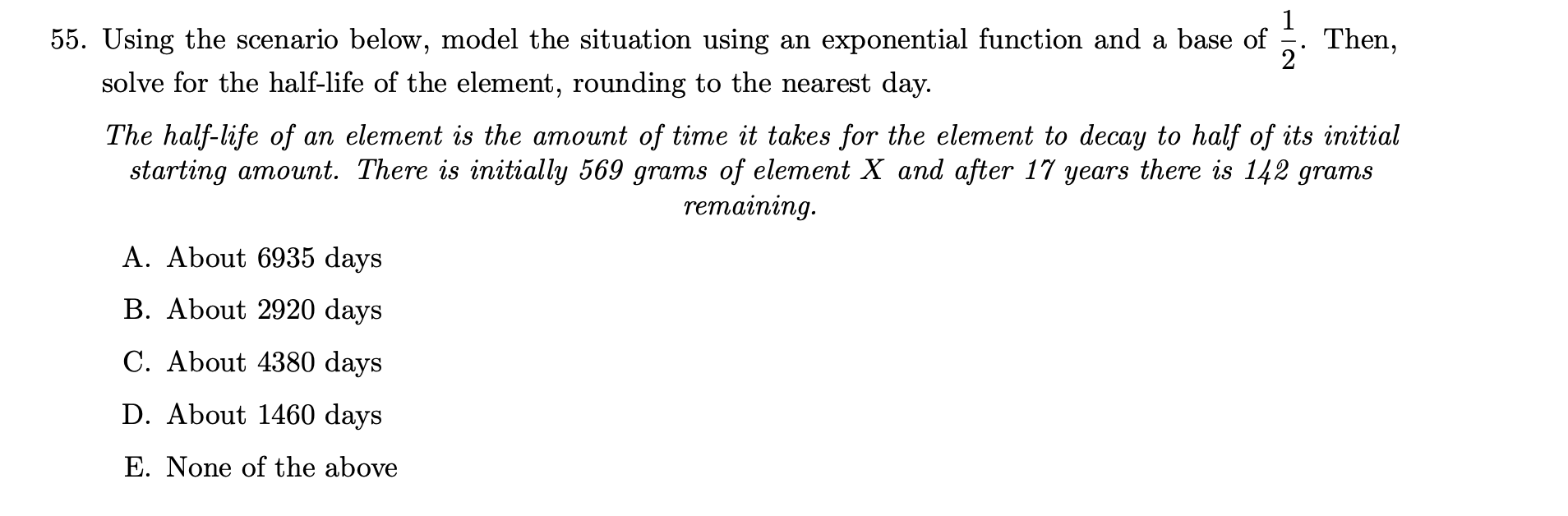1 55. Using the scenario below, model the situation using an exponential function and a base of Then, 2 solve for the half-life of the element, rounding to the nearest day. The half-life of an element is the amount of time it takes for the element to decay to half of its initial starting amount. There is initially 569 grams of element X and after 11 years there is 142 grams remaining A. About 6935 days B. About 2920 days...

• ### Answer the following questions regarding decay of Iridium-192 which is a first order reaction with a...

Answer the following questions regarding decay of Iridium-192 which is a first order reaction with a half life of 74 days Write the balanced equation representing Ir-192 undergoing beta decay and it accompanying rate law Determine the rate constant k for this reaction How many grams of a 50.0g sample of Iridium-192 remain after 5 years

• ### Modeling Exponential Growth and Decay A scientist begins with 240 grams of a radioactive substance. After...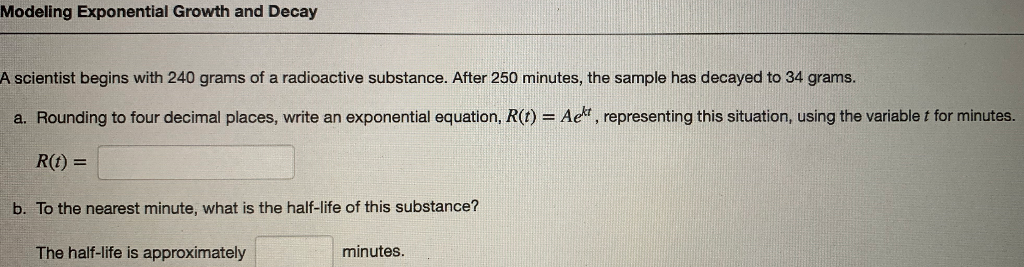Modeling Exponential Growth and Decay A scientist begins with 240 grams of a radioactive substance. After 250 minutes, the sample has decayed to 34 grams. a. Rounding to four decimal places, write an exponential equation, R(t) = Aekt, representing this situation, using the variablet for minutes. R(O) = b. To the nearest minute, what is the half-life of this substance? The half-life is approximately minutes.

• ### Strontium-90 has a half-life of 28 days. Using the exponential decay model Q(t) = Qoe-ht, find...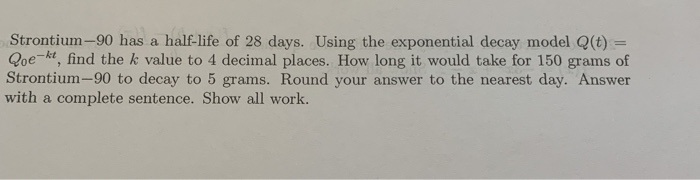Strontium-90 has a half-life of 28 days. Using the exponential decay model Q(t) = Qoe-ht, find the k value to 4 decimal places. How long it would take for 150 grams of Strontium-90 to decay to 5 grams. Round your answer to the nearest day. Answer with a complete sentence. Show all work.

• ### I have a test tomorrow and really need help seeing how to do numbers 1,2,3 and...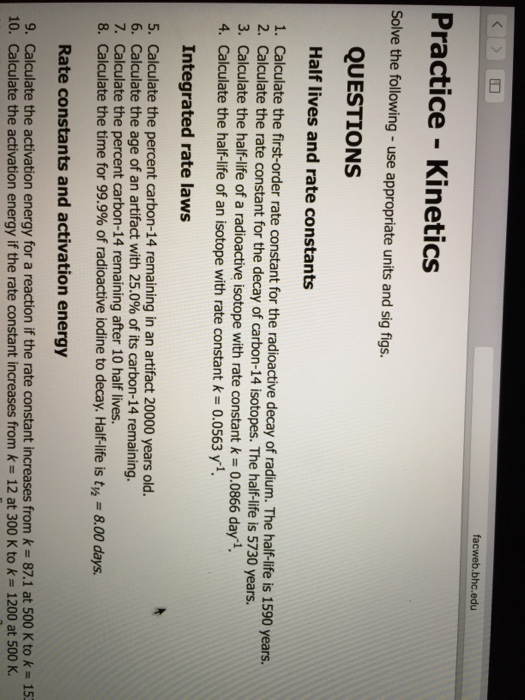I have a test tomorrow and really need help seeing how to do numbers 1,2,3 and 4. If you can start with telling me what equation to use, then solving using that equation, it would be much appreciated. facweb.bhc.edu Practice Kinetics Solve the following use appropriate units and sig figs. QUESTIONS Half lives and rate constants 1. Calculate the first-order rate constant for the radioactive decay of radium. The 1590 years. 2. Calculate the rate constant for the decay of...

• ### please show answer before and after rounding Finding the rate or time in a word problem...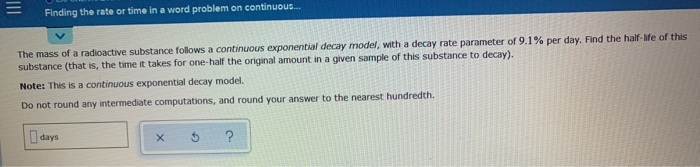please show answer before and after rounding Finding the rate or time in a word problem on continuous... The mass of a radioactive substance follows a continuous exponential decay model, with a decay rate parameter of 9.1% per day. Find the half-life of this substance (that is, the time it takes for one-half the original amount in a given sample of this substance to decay). Note: This is a continuous exponential decay model. Do not round any intermediate computations, and...

• ### 9. [-14 Points] DETAILS SPRECALC7 4.6.017. This exercise uses the radioactive decay model. The half-life of...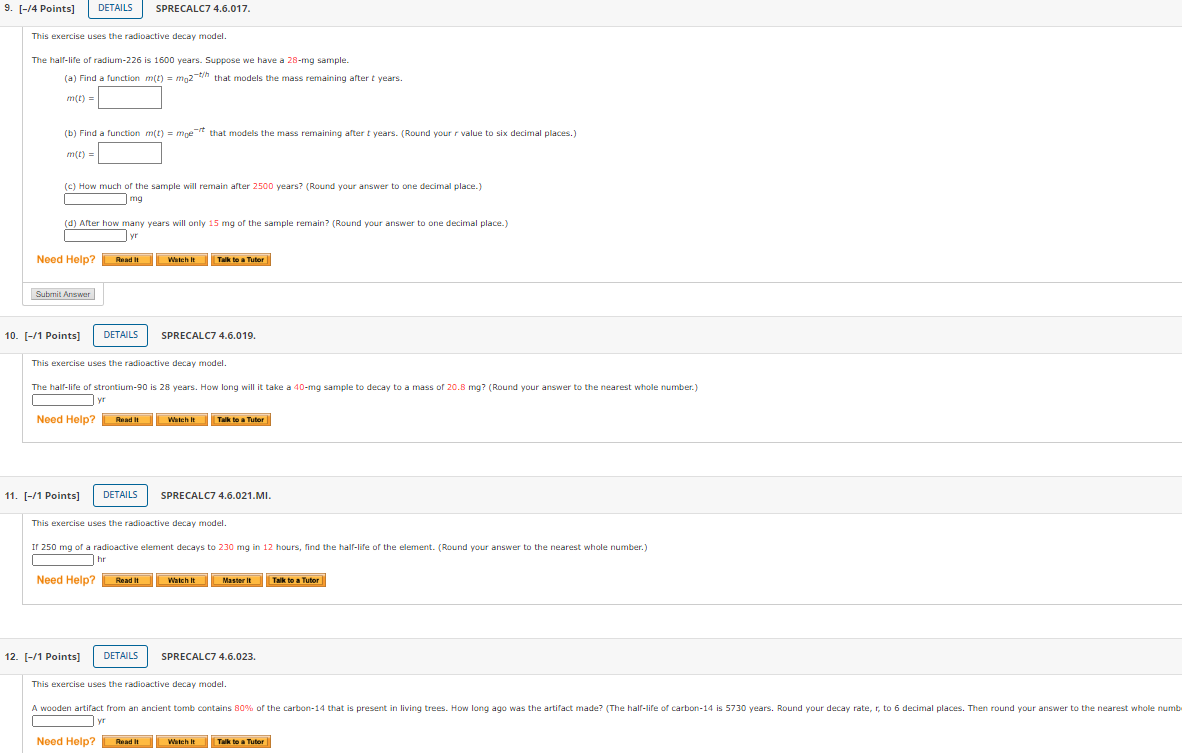9. [-14 Points] DETAILS SPRECALC7 4.6.017. This exercise uses the radioactive decay model. The half-life of radium-226 is 1600 years. Suppose we have a 28-mg sample. (a) Find a function m(t) = moz-th that models the mass remaining after t years. m(t) = (b) Find a function m(t) = moet that models the mass remaining after t years. (Round your value to six decimal places.) m(L) = (c) How much of the sample will remain after 2500 years? (Round your...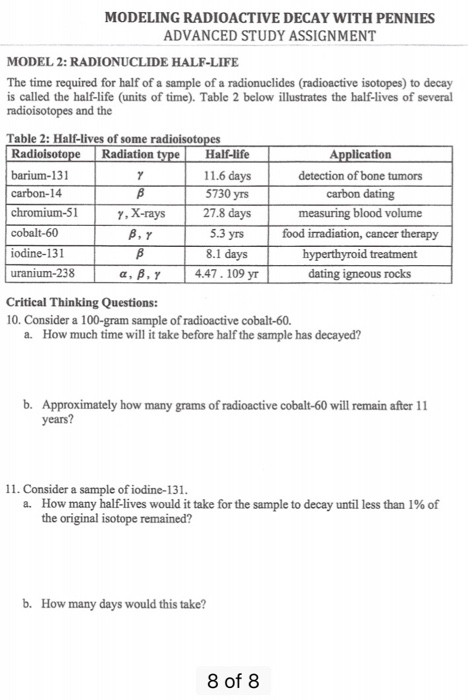Modeling radioactive decay in pennies MODELING RADIOACTIVE DECAY WITH PENNIES ADVANCED STUDY ASSIGNMENT MODEL 2: RADIONUCLIDE HALF-LIFE The time required for half of a sample of a radionuclides (radioactive isotopes) to decay is called the half-life (units of time). Table 2 below illustrates the half-lives of several radioisotopes and the Table 2: Half-lives of some Radioisoto barium-131 carbon-14 chromium-51 cobalt-60 iodine-131 uranium-238 Radiation Half-life 11.6 days 5730 yrs 27.8 days 5.3 yrs 8.1 days 4.47. 109 yr Application detection of...